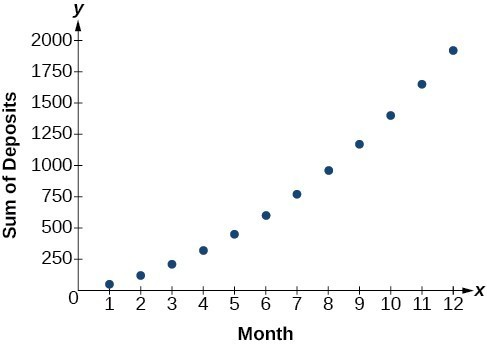## Solutions to Try Its

1. 38

2. $\text{26}\text{.4}$

3. $\text{328}$

4. $\text{-280}$

5. $2,025 6. $\approx 2,000.00$ 7. 9,840 8.$275,513.31

9. The sum is defined. It is geometric.

10. The sum of the infinite series is defined.

11. The sum of the infinite series is defined.

12. 3

13. The series is not geometric.

14. $-\frac{3}{11}$

15. $92,408.18 ## Solutions to Odd-Numbered Exercises 1. An $n\text{th}$ partial sum is the sum of the first $n$ terms of a sequence. 3. A geometric series is the sum of the terms in a geometric sequence. 5. An annuity is a series of regular equal payments that earn a constant compounded interest. 7. $\sum _{n=0}^{4}5n$ 9. $\sum _{k=1}^{5}4$ 11. $\sum _{k=1}^{20}8k+2$ 13. ${S}_{5}=\frac{5\left(\frac{3}{2}+\frac{7}{2}\right)}{2}$ 15. ${S}_{13}=\frac{13\left(3.2+5.6\right)}{2}$ 17. $\sum _{k=1}^{7}8\cdot {0.5}^{k - 1}$ 19. ${S}_{5}=\frac{9\left(1-{\left(\frac{1}{3}\right)}^{5}\right)}{1-\frac{1}{3}}=\frac{121}{9}\approx 13.44$ 21. ${S}_{11}=\frac{64\left(1-{0.2}^{11}\right)}{1 - 0.2}=\frac{781,249,984}{9,765,625}\approx 80$ 23. The series is defined. $S=\frac{2}{1 - 0.8}$ 25. The series is defined. $S=\frac{-1}{1-\left(-\frac{1}{2}\right)}$ 27.29. Sample answer: The graph of ${S}_{n}$ seems to be approaching 1. This makes sense because $\sum _{k=1}^{\infty }{\left(\frac{1}{2}\right)}^{k}$ is a defined infinite geometric series with $S=\frac{\frac{1}{2}}{1-\left(\frac{1}{2}\right)}=1$. 31. 49 33. 254 35. ${S}_{7}=\frac{147}{2}$ 37. ${S}_{11}=\frac{55}{2}$ 39. ${S}_{7}=5208.4$ 41. ${S}_{10}=-\frac{1023}{256}$ 43. $S=-\frac{4}{3}$ 45. $S=9.2$ 47.$3,705.42

49. $695,823.97 51. ${a}_{k}=30-k$ 53. 9 terms 55. $r=\frac{4}{5}$ 57.$400 per month

59. 420 feet

61. 12 feet